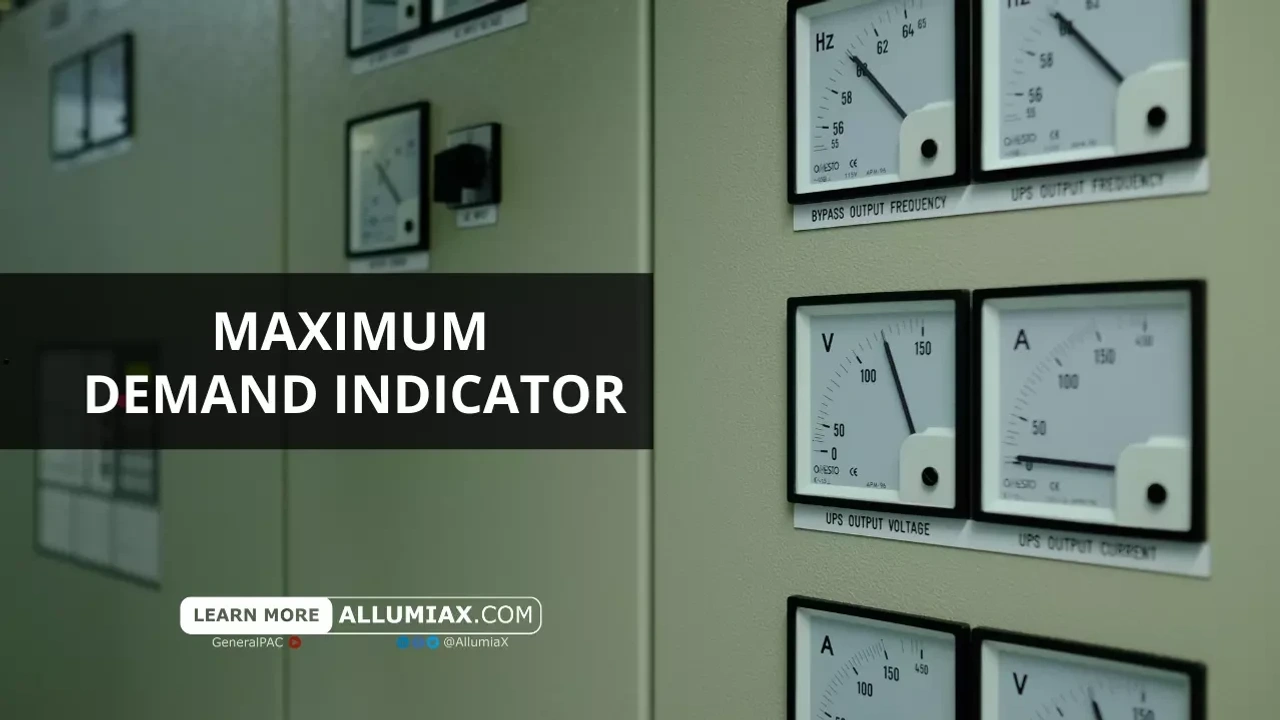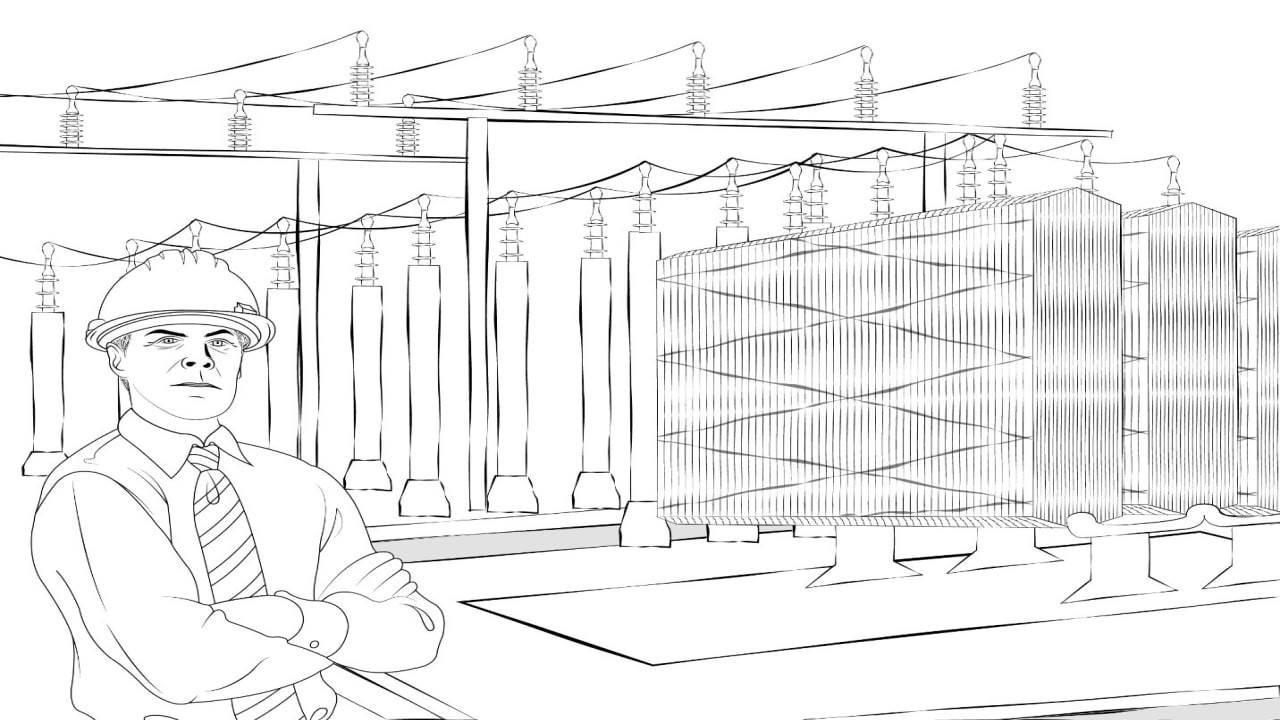(206) 687-4009 | [email protected]

# Maximum Demand Indicator (MDI)Last updated: May 30, 2022

It is essential for any power utility to provide sufficient energy enough for all the loads connected to the system. To achieve this, the generation and distribution system should be equipped with instruments to determine the maximum demand of consumers at all times. This can be done by the instrument called Maximum Demand Indicator (MDI). This blog describes the applications, pros and cons of different types of MDIs and the significance of calculating maximum demand.

## Maximum Demand

The greatest power demanded by load on the power station during a given time period is called maximum demand.

Maximum Demand =

Where,

• Load factor is the ratio of energy consumed in a given time period to the maximum demand in the specified time period. When the load factor value is low, then it indicates value of maximum demand to be very high. To improve load factor, electrical load operating at the peak time of the day can be shifted to off-peak hours.
• Average Load is the average of loads that occurred in a given time period also known as average demand.
Units generated during T hours in KWhTime (T) in hours

The demand charge for a given month is determined by the given formula:

Monthly Demand Charge (Cents)  =
KW of MD * demand charge in CentskW

### Significance of Maximum Demand:

Electricity billing structure by the utilities is usually composed of a two-part tariff structure, one part depends on the actual energy drawn (kWh), while other depends on the power demand (kVA or kW) during the billing cycle. Electric power suppliers charge commercial and industrial consumers such as industry, office buildings, hospitals etc. on the basis of maximum demand and total unit consumption. However, the residential customers are charged just for the “actual” energy (kW) used and not the “total” energy (kVA) supplied. With the increasing trends of energy conservation and cost saving, it is important for any consumer to understand the electricity tariffs.

Generally, utilities impose different demand charges for Peak and Off-Peak hour based on electricity billing structure. Let us explore the calculation of demand charges by an example.

Time of Use Time Charges

Peak time

5PM - 9PM

15.78 Cents/kW

Off peak time

remaining 20 hours

13.07 Cents/kW

Customer’s maximum demand during peak hours = 400 kW

Maximum demand during off-peak hours = 150 kW

Demand charges would be calculated as:

On-peak Demand: 400kW * 15.78 Cents = 6312 Cents

Off-peak Demand: 150kW * 13.07 Cents = 1960.5 Cents

Total Demand Charges for the month = On-peak Demand + Off-peak Demand= 6312+1960.5

Total Demand Charges for the month = 8272.5 Cents = \$ 82.725

Now assume another case in which peak demand is reduced during both on and off-peak hours:

Customer’s maximum demand during peak hours = 150 kW.

Maximum demand during off-peak hours = 300 kW.

Demand charges would be calculated as:

On-peak Demand: 150kW * 15.78 Cents = 2367 Cents

Off-peak Demand: 300kW * Cents. 13.07 = 3921 Cents

Total Demand Charges for the month = On-peak Demand + Off-peak Demand= 2367+3921

Total Demand Charges for the month = 6228 Cents = \$ 62.28

In the above example, we can observe a significant reduction in utility bill cost. Hence, we can conclude that when maximum demand is increased, higher will be the charges. There is need to reduce our maximum demand which is indicated by maximum demand indicator. So, maximum demand indicator holds great significance for utilities and consumers.

The importance of maximum demand lies in the fact that it helps in determining the installed capacity of the station and cost of installation. Higher the value of maximum demand on the station, greater will be the size and cost of installation. As the station capacity is optimized to meet the maximum demand. Now, low maximum demand means low capacity of the plant which, therefore, reduces the cost of the plant. Further, the tax charge and clerical staff depends upon the size of the plant and consequently, depend upon maximum demand.

## Maximum Demand Indicator:

Maximum Demand indicator is a device which records the maximum power used by the consumer at intervals. The indicator should only measure the base and peak load value not the high current values due to sudden short circuit or inrush current.

### Types of Maximum Demand Indicator:

Following are the Types of Maximum Demand Indicators:

1. Wright Maximum Demand Indicator
2. Merz-Price Demand Indicator

### Wright Maximum Demand Indicator:

Wright MDI is basically a thermal indicator operated by current. It works on the principle of differential thermometer. It is made up of U-shaped glass tube with two bulbs at upper ends of tube. The left side bulb consists of an air and metallic strip around it which is then connected with the load circuit in series. Beneath the right-side bulb, a scale is fitted along with a narrow bore Index tube. The main U-shaped tube is filled with dilute sulphuric acid, having low co-efficient of thermal expansion at low temperature. The traps in each limb prevents the movement of air from one bulb to the other.

The air inside the bulb expands when the load current flows through the strip. This expansion causes the indicator liquid of right-hand bulb to spill over into the index tube. The expansion of liquid highly depends on the magnitude of load current across the strip of the indicator. Thus, higher the magnitude of load current, the greater will be the expansion of liquid, and as a consequence, higher will be the quantity of liquid overflowing into the index tube. Now, the overflow of liquid will happen only when the load current through the strip exceeds the previous load current value.

For such indicator, time lag must be incorporated to prevent indication of peak demand of short duration. This time delay is increased by placing cast iron cylinder between bub and metallic strip (heater coil).

#### Applications of Wright Maximum Demand Indicator:

• It is commonly used in residential installations, due to its low cost as compared to Merz-price instrument.
• The Wright demand indicator can be used to determine the maximum loads on distribution transformer.

#### Advantages of Wright Maximum Demand Indicator:

• It is suitable for both D.C. and A.C. circuits.
• It can withstand heavy momentary overloads.
• The scale reads the number of amperes passing though coil only when that current is passing continuously for at least half an hour. It can be varied up to 3 hours.

#### Disadvantages of Wright Maximum Demand Indicator:

• The heat produced in the coil is proportional to the square of current flowing through it. Thus, the scale of the indicator is not uniform.
• The instrument gives the correct readings only at constant load power factor and supply voltage.

### Merz Price Indicator:

Merz Price Indicator is integrated with the energy meter which measures total energy consumed at particular time interval. The indicator records the maximum demand through a complex speed dial mechanism. Pointer connected to Merz demand indicator is driven by disc mechanism of energy meter using some gearing mechanism. Then, pin moves the dial forward which indicates the total power consumed at particular interval. For the next time interval, pointer will record the total power consumed by the load. The pin will move forward only when energy used by the load exceeds the previous recorded value. The indicator consists of cam and Bell crack mechanism, used to reset the pointer at initial position.

Merz Price indicator lies under the category of Average Demand Indicator as it shows the maximum value of the load averaged over equal time interval. The mathematical expression to calculate Average Maximum Demand is given as:

Average MD in kW =
Maximum Energy recorded (kWh)Time interval (h)

#### Advantages of Merz Price Indicator:

• It records peak load value with high accuracy.
• It has a complex mechanism but, the measuring scale is uniform.
• It can measure the power regarding kVARh or kVAh, by adding meter capable of measuring these quantities.

#### Disadvantages of Merz Price Indicator:

• The cost of average demand indicator is higher than Wright Demand Indicator due to complex gearing mechanism

Merz Price Indicator is of ultimate importance in Energy measurement field and is used as a unit together with an energy meter.

## Maximum Demand Penalty:

Once consumer specify the MD (Maximum Demand) needed during the month to utility, electric power supply company setup the generating station based on MD by load side. In an electrical system, an increase in the demand for electricity by more diverse end user leads to shortfall in capacity to meet demand. To keep up with energy demands, the construction of new generation power plants are costly as well as a long wait prospective. So, utility imposes a penalty on consumer if MD value during the month is higher than the contracted demand. This penalty is accounted in their electricity bill and is termed as MD Penalty.

### Ways to avoid MD Penalty:

MD penalties are incurred by utility because of exceeding the contracted power can be reduced by practicing the following methods:

1. #### Power Factor Improvement:

Penalties can be avoided by usage of electrical appliances that give more output (kW) per kVA improve the power factor efficiency. PF can be improved by adding PF correction capacitors to the electrical system, which act as reactive current generators to the inductive load. Thus, reducing the power losses, and alternatively contracted MD does not exceed.

👉🏼 We previously wrote a blog on Power Factor. Go through the article to get more information about power factor correction techniques.

2. #### Peak Shaving of Load Curves:

MDI penalty can be avoided by smartly managing loads according to billing structure. This can be done by levelling of the daily load curve i.e., shifting the peak loads to a time when connected load are of low demand, by limiting the consumption during the day when the tariffs of the electrical energy are higher. Moreover, the consumers can gain visibility to their energy data by real-time monitoring, which will display when the demand for electricity is high and will allow the consumer to change methods for cutting those peaks, also the demand charges.
3. #### Load Management using Time of Use (TOU) Method:

Better load management on the user side minimizes the peak demands on the utility infrastructures, thus improves the utilization of power plant capacities. MD controllers, MD panel and power controller can be used to limit the average power consumption to a contracted power (pre-determined maximum value). MD controllers for load management during peak hours curtail the non-vital loads when the demand exceeds the pre-defined load limit.

As MD contributes to the considerable portion of the electricity bill cycling, to effectively control the maximum demand during the month, there is a real need of integrating Power Demand Controller. The gap of supply and demand have compelled power engineers to develop techniques for Demand Side Management (DSM) and Load Management such that utility and consumers can make the most of electric energy. MD controllers based on novel techniques have been implemented and proved that managing loads on peak hours increases the reliability of power system by lowering the power cuts.

Close